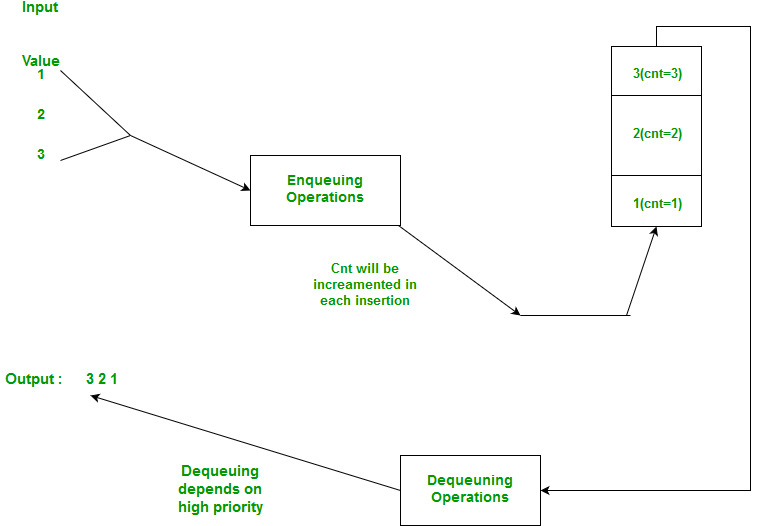Open in App
Not now

# How to implement stack using priority queue or heap?

• Difficulty Level : Medium
• Last Updated : 31 Jan, 2023

How to Implement stack using a priority queue(using min heap)?. Asked In: Microsoft, Adobe.

Solution: In priority queue, we assign priority to the elements that are being pushed. A stack requires elements to be processed in Last in First Out manner. The idea is to associate a count that determines when it was pushed. This count works as a key for the priority queue. So the implementation of stack uses a priority queue of pairs, with the first element serving as the key.

## CPP

 `pair& lt;``int``, ``int``& gt;``(key, value)`

See Below Image to understand BetterBelow is C++ implementation of the idea.

## C++

 `// C++ program to implement a stack using``// Priority queue(min heap)``#include``using` `namespace` `std;` `typedef` `pair<``int``, ``int``> pi;` `// User defined stack class``class` `Stack{``    ` `    ``// cnt is used to keep track of the number of``    ``//elements in the stack and also serves as key``    ``//for the priority queue.``    ``int` `cnt;``    ``priority_queue > pq;``public``:``    ``Stack():cnt(0){}``    ``void` `push(``int` `n);``    ``void` `pop();``    ``int` `top();``    ``bool` `isEmpty();``};` `// push function increases cnt by 1 and``// inserts this cnt with the original value.``void` `Stack::push(``int` `n){``    ``cnt++;``    ``pq.push(pi(cnt, n));``}` `// pops element and reduces count.``void` `Stack::pop(){``    ``if``(pq.empty()){ cout<<``"Nothing to pop!!!"``;}``    ``cnt--;``    ``pq.pop();``}` `// returns the top element in the stack using``// cnt as key to determine top(highest priority),``// default comparator for pairs works fine in this case``int` `Stack::top(){``    ``pi temp=pq.top();``    ``return` `temp.second;``}` `// return true if stack is empty``bool` `Stack::isEmpty(){``    ``return` `pq.empty();``}` `// Driver code``int` `main()``{``    ``Stack* s=``new` `Stack();``    ``s->push(1);``    ``s->push(2);``    ``s->push(3);``    ``while``(!s->isEmpty()){``        ``cout<top()<pop();``    ``}``}`

## Java

 `// Java program to implement a stack using``// Priority queue(min heap)``import` `java.util.PriorityQueue;` `class` `Stack``{` `  ``// cnt is used to keep track of the number of``  ``//elements in the stack and also serves as key``  ``//for the priority queue.``  ``int` `cnt;``  ``PriorityQueue<``int``[]> pq = ``new` `PriorityQueue<>((a, b) -> a[``0``] - b[``0``]);` `  ``public` `Stack() {``    ``cnt = ``0``;``  ``}` `  ``public` `void` `push(``int` `n) {``    ``cnt++;``    ``pq.offer(``new` `int``[]{cnt, n});``  ``}` `  ``public` `void` `pop() {``    ``if` `(pq.isEmpty()) {``      ``System.out.println(``"Nothing to pop!!!"``);``      ``return``;``    ``}``    ``cnt--;``    ``pq.poll();``  ``}` `  ``public` `int` `top() {``    ``int``[] temp = pq.peek();``    ``return` `temp[``1``];``  ``}` `  ``public` `boolean` `isEmpty() {``    ``return` `pq.isEmpty();``  ``}` `  ``public` `static` `void` `main(String[] args) {``    ``Stack s = ``new` `Stack();``    ``s.push(``3``);``    ``s.push(``2``);``    ``s.push(``1``);``    ``while` `(!s.isEmpty()) {``      ``System.out.println(s.top());``      ``s.pop();``    ``}``  ``}``}` `// This code is contributed by adityamaharshi21`

## Python3

 `import` `heapq` `# User defined stack class``class` `Stack:``    ``# cnt is used to keep track of the number of``    ``# elements in the stack and also serves as key``    ``# for the priority queue.``    ``def` `__init__(``self``):``        ``self``.cnt ``=` `0``        ``self``.pq ``=` `[]` `    ``def` `push(``self``, n):``        ``# push function increases cnt by 1 and``        ``# inserts this cnt with the original value.``        ``self``.cnt ``+``=` `1``        ``heapq.heappush(``self``.pq, (``-``self``.cnt, n))` `    ``def` `pop(``self``):``        ``# pops element and reduces count.``        ``if` `not` `self``.pq:``            ``print``(``"Nothing to pop!!!"``)``        ``self``.cnt ``-``=` `1``        ``return` `heapq.heappop(``self``.pq)[``1``]` `    ``def` `top(``self``):``        ``# returns the top element in the stack using``        ``# cnt as key to determine top(highest priority),``        ``# default comparator for pairs works fine in this case``        ``return` `self``.pq[``0``][``1``]` `    ``def` `isEmpty(``self``):``        ``# return true if stack is empty``        ``return` `not` `bool``(``self``.pq)` `# Driver code``s ``=` `Stack()``s.push(``1``)``s.push(``2``)``s.push(``3``)``while` `not` `s.isEmpty():``    ``print``(s.top())``    ``s.pop()`

## Javascript

 `class Stack {``    ``constructor() {``        ``this``.stack = [];``    ``}` `    ``push(n) {``        ``this``.stack.push(n);``    ``}` `    ``pop() {``        ``if` `(``this``.stack.length === 0) {``            ``console.log(``"Nothing to pop!!!"``);``        ``}``        ``return` `this``.stack.pop();``    ``}` `    ``top() {``        ``return` `this``.stack[``this``.stack.length - 1];``    ``}` `    ``isEmpty() {``        ``return` `this``.stack.length === 0;``    ``}``}` `// Driver code``let s = ``new` `Stack();``s.push(1);``s.push(2);``s.push(3);``while` `(!s.isEmpty()) {``    ``console.log(s.top());``    ``s.pop();``}`

Output

```3
2
1```

Time Complexity: O(logn)

Now, as we can see this implementation takes O(log n) time for both push and pop operations. This can be slightly optimized by using fibonacci heap implementation of priority queue which would give us O(1) time complexity for push operation, but pop still requires O(log n) time.

Auxiliary Space: O(n) where n is size of priority queue